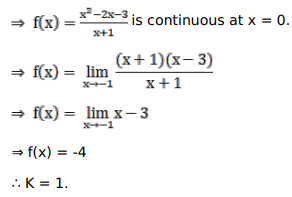# Solve this following

Question:

Mark $(\sqrt{)}$ against the correct answer in the following:

Let $f(x)=\left\{\begin{array}{r}\frac{x^{2}-2 x-3}{x+1^{2}}, \text { when } x \neq-1 \\ k, \text { when } x=-1\end{array}\right.$

If $f(\mathrm{x})$ is continuous at $\mathrm{x}=-1$ then $\mathrm{k}=?$

A. 4

B. $-4$

C. $-3$

D. 2

Solution: